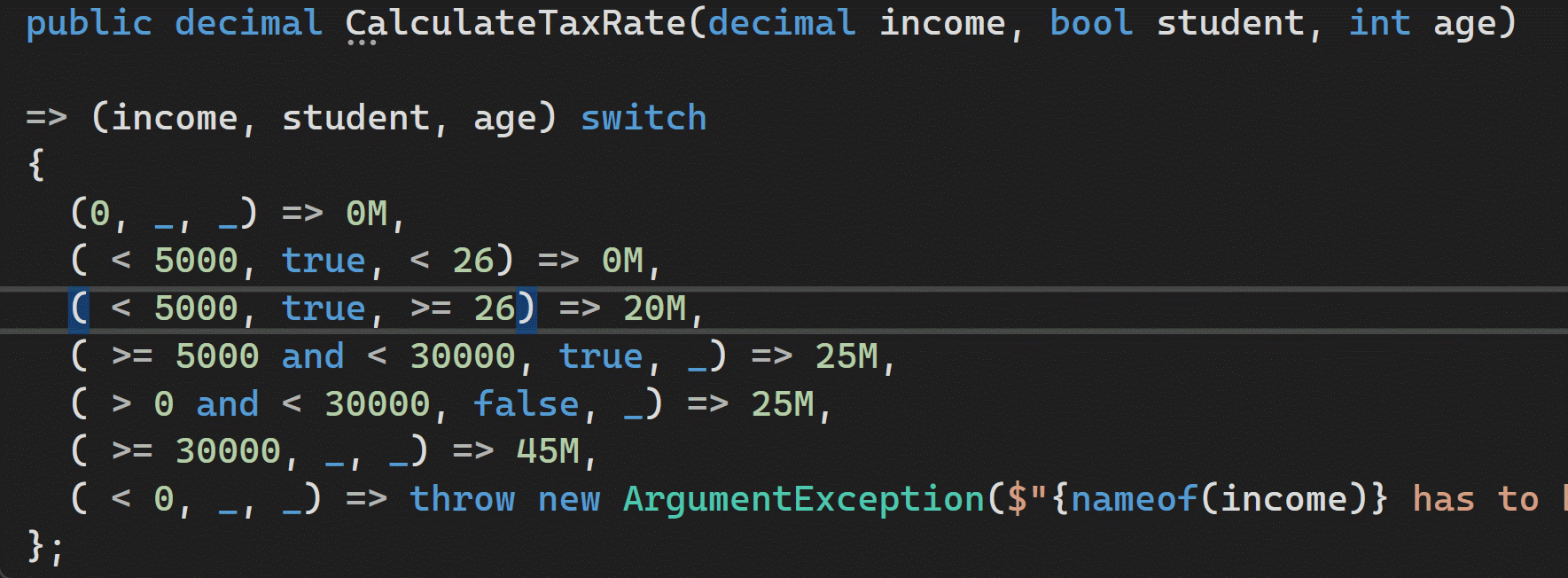Complex business logic can be simplified by implementing this as a decision table using C# pattern matching.

## What are Decision Tables?

Decision tables are an excellent tool to model complex business logic, and give you a straightforward way to detect missing combinations which should also be considered.

Below I have a simple decision table to calculate taxes based on age and income.

This example is fictitious!

• When your income is 0, you pay 0% taxes.
• As a student you are allowed earn up to 5000 without paying taxes, on the condition you are less than 26 years old.
• Otherwise, older students pay 20% taxes up to 5000.
• Students who make more than 5000 pay 25%.
• All other people pay 25% if their income does not exceed 30000.
• When you earn more than 30000 you pay 45% taxes, even students.
• Negative incomes are not possible.
``````+-----------------------------------------------+
|  income   |  student |    age   | tax rate    |
+-----------------------------------------------+
|     0     |          |          |     0       |
+-----------------------------------------------+
| <   5000  |   true   |  <  26   |     0       |
+-----------------------------------------------+
| <   5000  |   true   |  >= 26   |    20       |
+-----------------------------------------------+
| >=  5000  |   true   |          |    25       |
| <  30000  |          |          |             |
+-----------------------------------------------+
| >      0  |   false  |          |    25       |
| <  30000  |          |          |             |
+-----------------------------------------------+
| >= 30000  |          |          |    45       |
+-----------------------------------------------+
| <      0  |          |          |    45       |
+-----------------------------------------------+
``````

## Using C# Pattern Matching to Implement Decision Tables

C# has support for pattern matching which allows you to take a bunch of values and use combinations of these in logical expressions which are clearer and shorter then the equivalent `if` statements.

I have implemented the above decision table in the `CalculateTaxRate` method below.

``````public decimal CalculateTaxRate(decimal income, bool student, int age)

=> (income, student, age) switch
{
(0                   , _    , _    ) => 0M,
( < 5000             , true , < 26 ) => 0M,
( < 5000             , true , >= 26) => 20M,
( >= 5000 and < 30000, true , _    ) => 25M,
( > 0 and < 30000    , false, _    ) => 25M,
( >= 30000           , _    , _    ) => 45M,
( < 0                , _    , _    ) => throw new ArgumentException(\$"{nameof(income)} has to be at least 0")
};
``````

This code matches the decision table in a perfect way!

Using a `switch` expression we can implement each row of the decision table.

• Empty cells in the decision table are implemented using a discard expression `_`.
• Constant patterns such as `0` and `true` are used to check for equality.
• Relational patterns such as `< 5000` are used to compare values.
• Logical patterns such as `and`, `or` and `not` can be used to combine expressions.